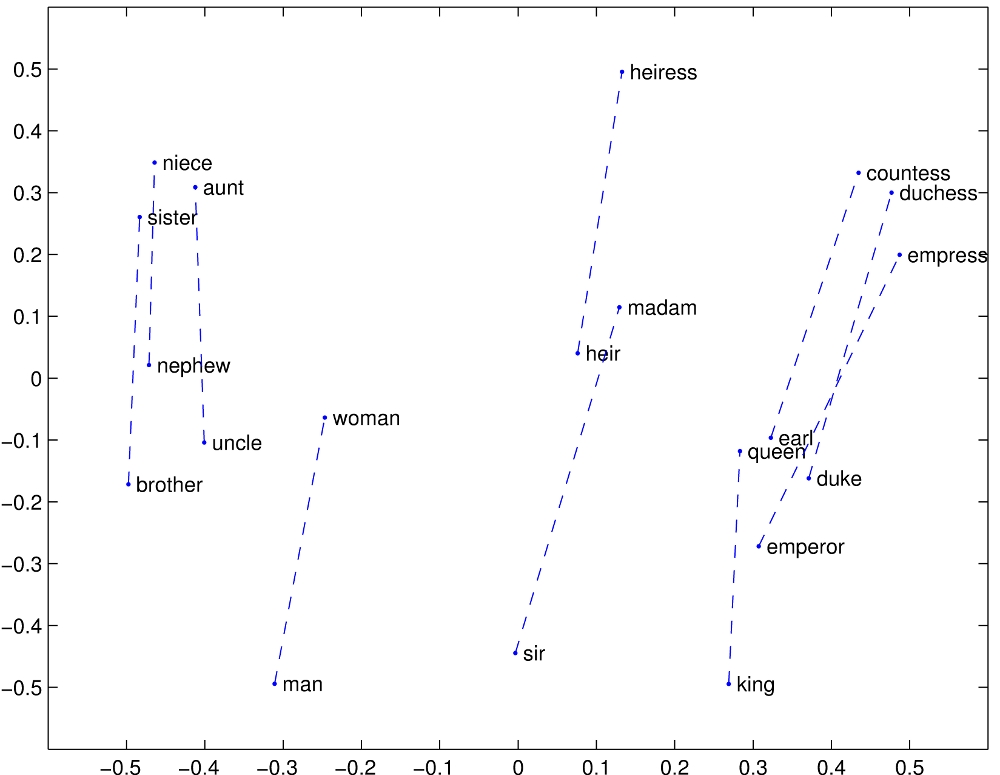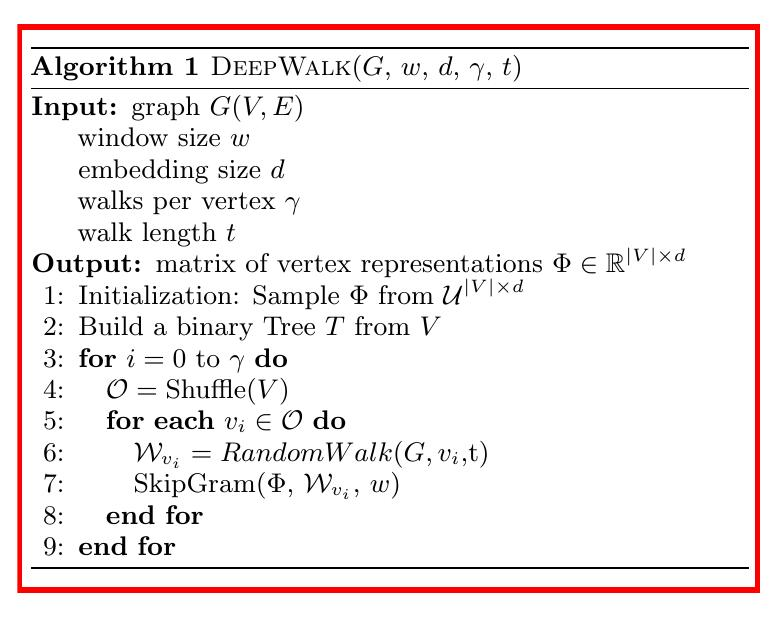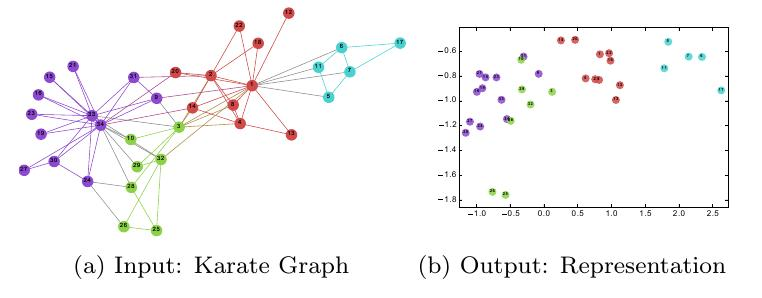# Demystify DeepWalk and Crack Its Code

## Brief Overview of DeepWalk

Posted by Chi Zhang on May 9, 2016

## 1. Introduction

The algorithm of DeepWalk  was first introduced by Bryan Perozzi, Rami Al-Rfou and Steven Skiena from Stony Brook University.

In a word, DeepWalk tries to learn good latent representations, or in Natural Language Processing terminology, embeddings, of vertices in a graph by local information obtained by truncated random walks.

Then how they did it?

## 2. Motivation

Their motivation actually comes from the wide application of the advanced language models that were first used to extract, unsupervisedly from raw texts, latent distributed representations for words.

#### 2.1. Distributed Representation

To help you better understand what I mean by distributed, let me show you an example.

Prior to the distributed representations, folks normally applied one-hot embeddings to encode words.

For example, suppose there are 6 words in your corpus, “boy”, “girl”, “prince”, “princess”, “king” and “queen”. One-hot representation encodes each word by a vector which has a single one at a predefined location.

Word Embedding
boy $(1, 0, 0, 0, 0, 0)$
girl $(0, 1, 0, 0, 0, 0)$
prince $(0, 0, 1, 0, 0, 0)$
princess $(0, 0, 0, 1, 0, 0)$
king $(0, 0, 0, 0, 1, 0)$
queen $(0, 0, 0, 0, 0, 1)$

Table 1. One-hot representation.

However, these representations do not encode any useful information about the semantics and syntactics of the words.

So, let’s do it in a different way.

Actually, there is no oracle that says the embedding must contain a single nonzero number in a fixed position. So as Yoshua Bengio  proposed, why not use a arbitrary vector to represent a word?

Like, why couldn’t “boy” be encoded $(0.12, 3.42, 0.012, 9.18, 14.00, -0.25)$?

Note how it all changes the way we see words computationally. This idea opens up a whole N-dimensional space for us to represent each word. And as you might expect, semantics and syntactics could now manifest themselves as clusters in the space.

But then comes another question – how do we obtain such embeddings?

#### 2.2. Neural Language Model

An explanation of word embeddings is not complete without reference to this paper  from Tomas Mikolov. In this paper, researchers proposed an efficient algorithm to learn the embeddings aforementioned. They introduced two independent algorithms, CBOW and SkipGram. But since only SkipGram relates to the work of DeepWalk, I will simply leave the explanation of CBOW to the future.

Formally, SkipGram tries to minimize the negative joint log probability of its context given the embedding of the center word,

where $w$ is half the size of the window that surrounds the center.

By modelling a probabilistic neural language model, or a 2-layer neural network that independently predicts the distribution of surrounding words and minimizing the log-prob, we could learn very powerful and meaningful embeddings.Figure 1. Visualizing word vectors. Notice that words with similar semantics are clustered together.

#### 2.3. Random Walk

The only essential compartment left to be explained is the concept of random walk. The phrase of random walk is usually encountered in literature of stochastic process. It basically describes a process of transferring randomly among different states or graph nodes.

Suppose you’re in a node $n$ of a graph $G(V, E)$. The node has $m$ neighbors. Then, in a completely random walk, you transfer to any of your neighbor with a probability of $1 \over m$.

Well, I’ve covered all the basics and now let’s move to the algorithm itself.

## 3. Putting All Together

The algorithm bears the resemblance to Natural Language Processing in that it treats each node as a word in a sequence, which in the algorithm is produced by the random walk, hence the name DeepWalk.

The entire algorithm is illustrated as follows:In the algorithm, the authors built a binary hierarchical softmax tree to help select words, but since this is only a minor component and the paper has a easy-to-understand writeup for this section, I decide to leave it to the readers to find in the paper.Figure 2. Visualizing DeepWalk. The left plot shows the ground truth while the right demonstrates effective embeddings.

## 4. DeepWalk Implementation

To help thoroughly understand how the algorithm crunches the data, one should definitely read the source code.

In fact, there have already been a variety of implementations of the algorithm by different deep learning frameworks, including Tensorflow and Keras on GitHub. I would personally suggest the original repository  by the authors since it not only retains the source ideas but also shows minimum dependency on other packages. I also have a personal fork  that provide a near-complete comments on the formatted source code.

There are several source files in the deepwalk directory.

File Function
__init__.py Package info
__main__.py Main algorithm
graph.py Utilities for the graph structure
skipgram.py Wrapper for Word2Vec
walks.py Corpus generation

Table 2. DeepWalk codebase.

The highest level abstraction is pretty straightforward: generate walks then train.

print("Walking...")
walks = graph.build_deepwalk_corpus(G, num_paths = args.number_walks,
path_length = args.walk_length, alpha = 0,
rand = random.Random(args.seed))
print("Training...")
model = Word2Vec(walks, size = args.representation_size,
window = args.window_size, min_count = 0,
workers = args.workers)


However, details are explained in the corresponding files. If you find it a bit hard to understand the code, feel free to access my fully-commented fork here .

## 5. Conclusion

DeepWalk has made great contribution in the field of network learning and community-based data mining due to its accuracy and scalability.

With the latent representations, people could easily leverage the hidden knowledge in the networks to model similarities among nodes in the graph, making a huge boost in performance for data-related tasks.

If everything goes well, I will update our work on how DeepWalk contributes to knowledge discovery in the near future. So stay tuned!